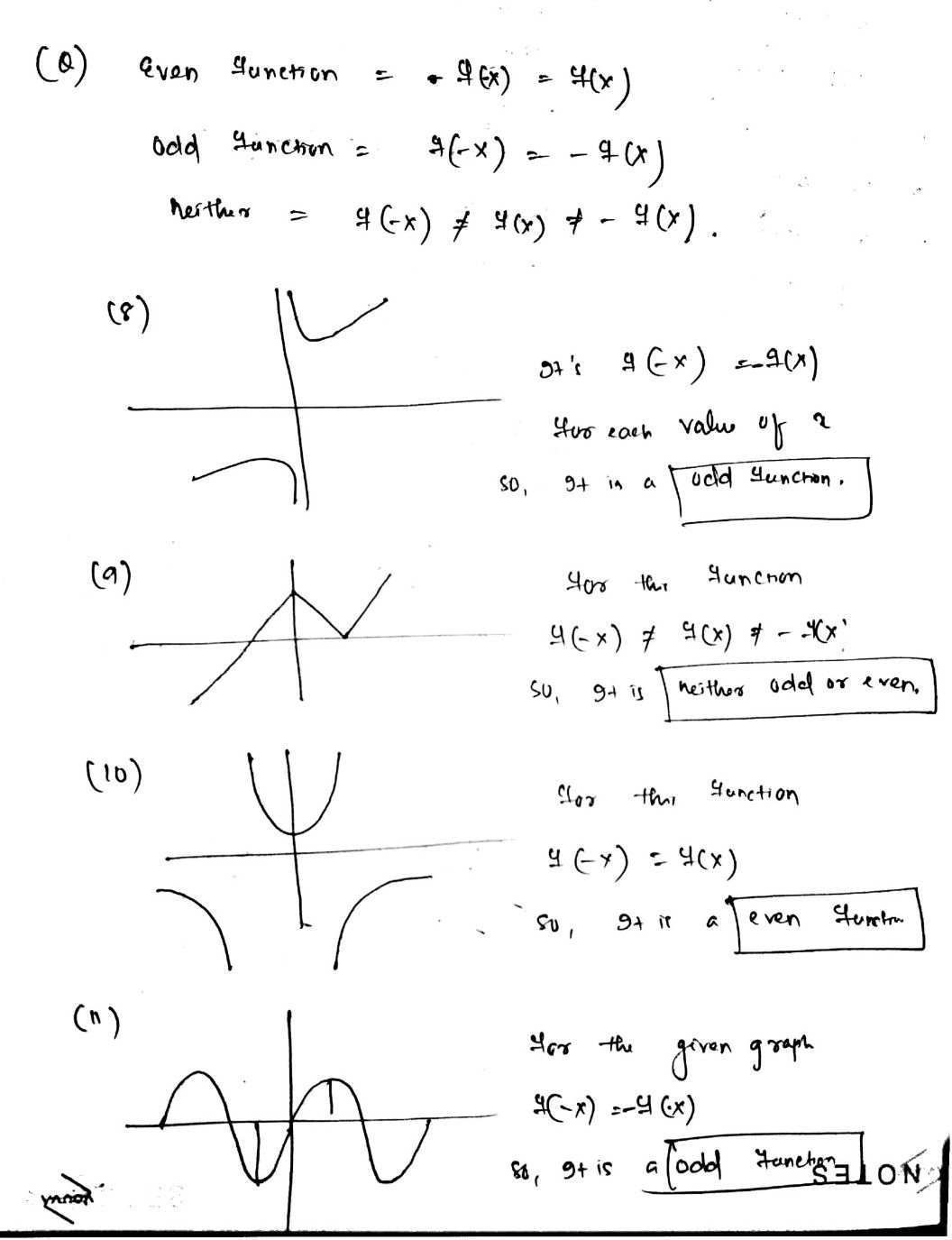Symbol
ProblemDetermine whether each function is even, odd, or neither. Explain. 8. 9. $4^{1}$ $-3$ $\vec{5x}$ $-5$ $\square$ Scanned with CamScanner $C$ Scanned $0$ $00$ $00n2$ $10$ $11$ $Q$ $0$ $\left(-\dfrac {4,0} {-3}\right)\right)$ $+$ $\left(,°$ $3^{x0}$ -1 $\sqrt{}$ $2$ $+$ $∩$ $-5-$ hned with CamScanner $0=$
Calculus
Search count: 107
SolutionQanda teacher - abikshyanaplease evaluate the answer and give gift coins please ☺️☺️ for giving gift coins Click on evaluate. Then press the + icon 3 times(3000).